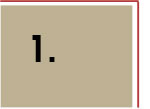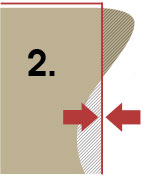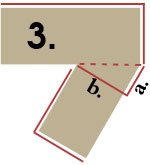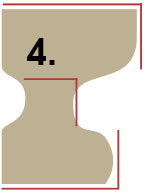# Calculating Irregular Areas

### Calculating Irregular Areas

These may not offer perfect calculations but will give you a more accurate cubic yard measurement when ordering topsoil.

 Square Area Calculation This is the standard method shown in STEP 1 above. Length x WidthCurved Side Area Move your measurement left or right along the curve to balance the area to be covered with the area that is not to be covered. If this is still too difficult to judge, follow guideline for Odd Shapes shown below. Length x WidthJoined Shapes Areas Visually divide the area to be covered into parts for easier measurement. As shown here, dividing this area into three parts makes things easier. In this case, measure the two rectangles and one triangle, then add them together. Length x Width for top rectangle area PLUS Length x Width for bottom rectangle area PLUS Length(b.) x Width(a.) ÷ 2 for the triangle areaOdd Shape Areas Try to find square shapes within an odd shape and add them together. In this example, there are three squares found in an odd shape. Length x Width for top rectangle area PLUS Length x Width for center rectangle area PLUS Length x Width for bottom rectangle areaReference for calculations and page content http://backwoodsexcavating.com/calculate-cubic-yards.php